# Colleges with the highest SAT scores in Virginia

Top 10 colleges in Virginia with the highest SAT scores
Looking for the colleges with the highest SAT scores in Virginia? Well you're in luck! We've compiled a national college database and have created a list of the top 10 universities with the highest SAT scores in Virginia. These are the schools whose applicants had the highest average SAT scores in Virginia. And, since these tests are meant to determine academic prowess, they are arguably the schools with the most academically proficient students. You could even say these are the best colleges in Virginia. We also include each college's ACT scores and acceptance rate so that you can see where you would have the easiest or hardest time getting in. Read on to find out more.

## University of Virginia SAT scores

The average SAT score for University of Virginia is 1430.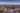The average SAT score of 1430 breaks down into:

• SAT math: 725

The average ACT score for University of Virginia is 32 and their acceptance rate is 23.9%.

## Washington and Lee University SAT scores

The average SAT score for Washington and Lee University is 1420.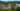The average SAT score of 1420 breaks down into:

• SAT math: 720

The average ACT score for Washington and Lee University is 32 and their acceptance rate is 21.2%.

## The College of William and Mary SAT scores

The average SAT score for The College of William and Mary is 1415.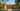The average SAT score of 1415 breaks down into:

• SAT math: 715

The average ACT score for The College of William and Mary is 32 and their acceptance rate is 37.7%.

## University of Richmond SAT scores

The average SAT score for University of Richmond is 1375.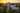The average SAT score of 1375 breaks down into:

• SAT math: 700

The average ACT score for University of Richmond is 32 and their acceptance rate is 30.2%.

## Virginia Polytechnic Institute and State University SAT scores

The average SAT score for Virginia Polytechnic Institute and State University is 1285.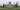The average SAT score of 1285 breaks down into:

• SAT math: 650

The average ACT score for Virginia Polytechnic Institute and State University is 28 and their acceptance rate is 64.8%.

## Christendom College SAT scores

The average SAT score for Christendom College is 1260.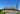The average SAT score of 1260 breaks down into:

• SAT math: 600

The average ACT score for Christendom College is 26 and their acceptance rate is 88.8%.

## George Mason University SAT scores

The average SAT score for George Mason University is 1215.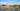The average SAT score of 1215 breaks down into:

• SAT math: 605

The average ACT score for George Mason University is 27 and their acceptance rate is 86.7%.

## James Madison University SAT scores

The average SAT score for James Madison University is 1205.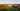The average SAT score of 1205 breaks down into:

• SAT math: 595

The average ACT score for James Madison University is 26 and their acceptance rate is 71.1%.

## Christopher Newport University SAT scores

The average SAT score for Christopher Newport University is 1203.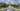The average SAT score of 1203 breaks down into:

• SAT math: 590

The average ACT score for Christopher Newport University is 26 and their acceptance rate is 67.8%.

## University of Mary Washington SAT scores

The average SAT score for University of Mary Washington is 1172.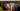The average SAT score of 1172 breaks down into:

• SAT math: 570